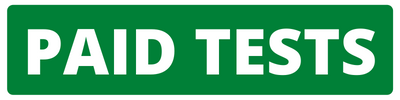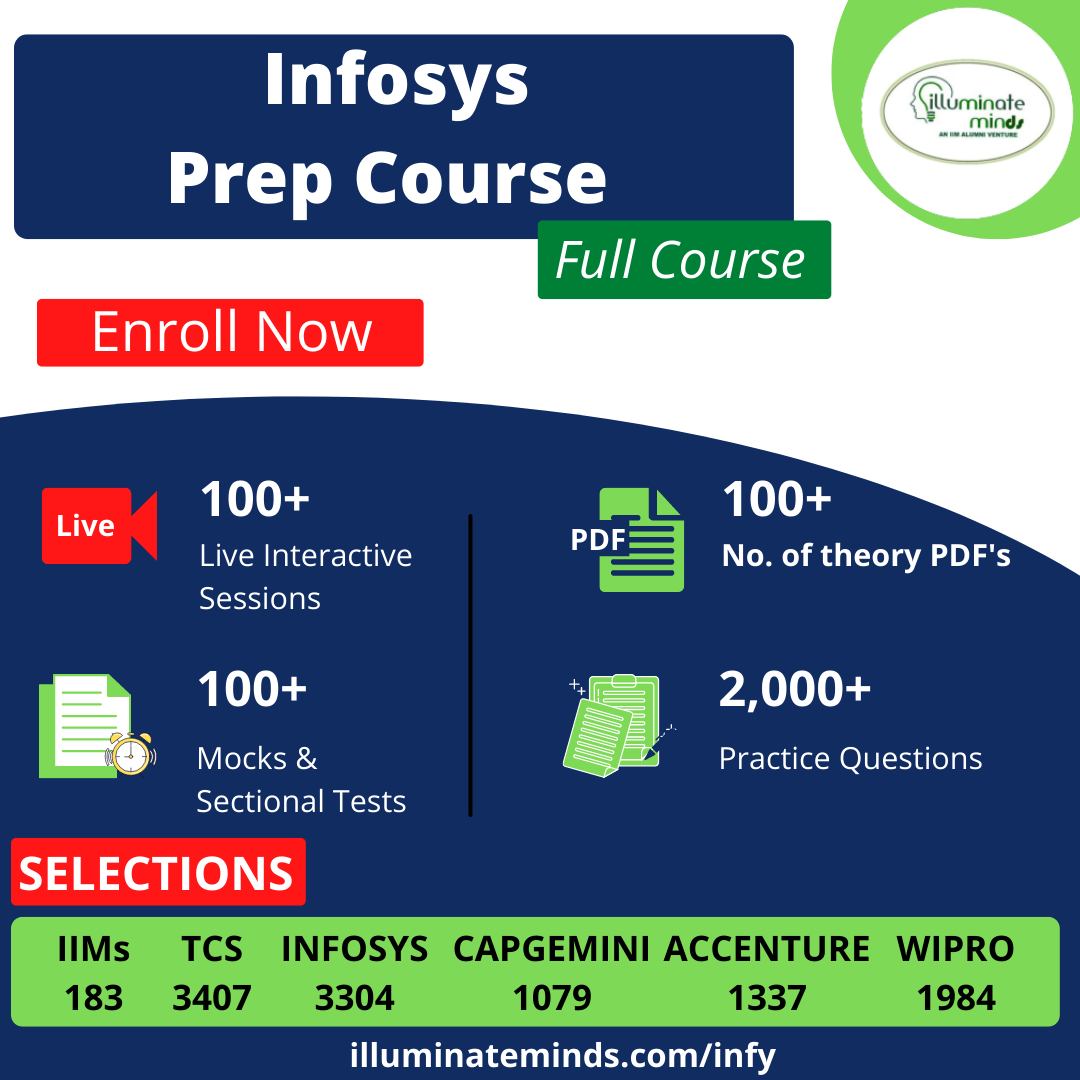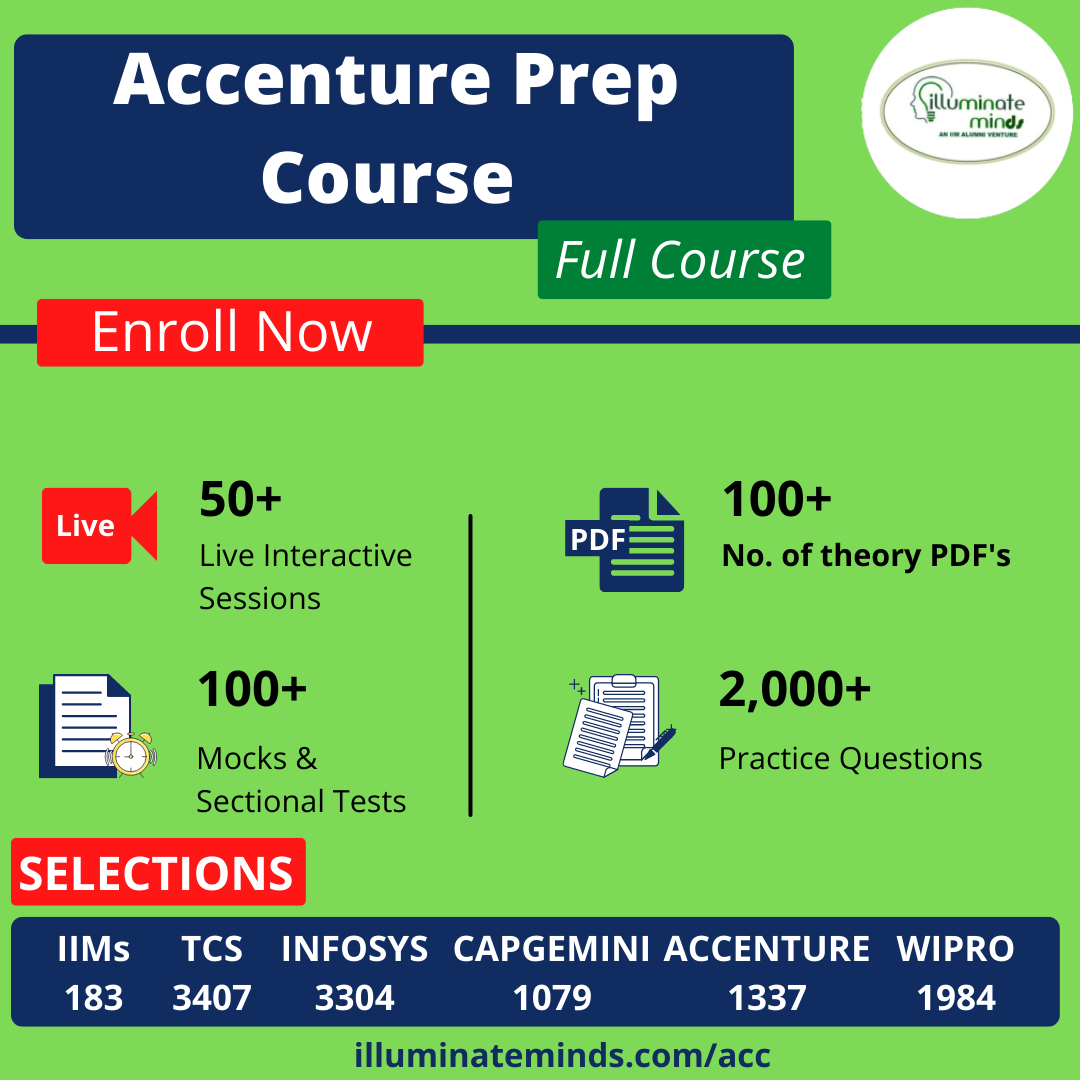TCS ION CCQT Quants Questions

## TCS ION CCQT Quants QuestionsJobs By Batch
Jobs By Location
Jobs By Degree
Jobs By Branch
IT Jobs
Internships
Govt. Jobs• ##### Original Questions of IT Companies| All Topics### Quiz Begins Here

Q #1
:

#### Quants Question

Directions: Read the following information to answer the question.

Three times the first of three consecutive odd integers is 3 more than twice the third. The third integer is:
+

Let the three integers be x, x + 2 and x + 4.

Then, 3x = 2(x + 4) + 3      x = 11.

Third integer = x + 4 = 15.

Q #2
:

#### Quants Question

Directions: Read the following information to answer the question.

If 4 (P's capital) = 6 (Q's capital) = 10 (R's capital), then out of a profit of Rs. 4650, R will receive ____
+

Let 4 = 6Q = 1OR = k. Then, P = k/4, Q = k/6, and R =k/10.

P : Q :R = k/4 : k/6 : k/10 = 15 : 10 : 6.

Hence, R's share (4650 * 6/31) = Rs, 900.

Q #3
:

#### Quants Question

Directions: Read the following information to answer the question.

Successive discounts of 20% and 15% are equivalent to a single discount of:
+

 Reqd single discount= (100 – 100 ×80/100×85/100)% = 32%

Q #4
:

#### Quants Question

Directions: Read the following information to answer the question.

The simple interest on a certain sum of money at the rate of 5% p.a. for 8 years is Rs. 840. At what rate of intrest the same amount of interest can be received on the same sum after 5
+

Here firstly we need to calculate the principal amount, then we can calculate the new rate.

P=S.I.∗100R∗TP=840∗1005∗8P=2100Required Rate = 840∗1005∗2100R=8%

Q #5
:

#### Quants Question

Directions: Read the following information to answer the question.

If P > 5, q > - 2, which of the following is necessarily true?
+

Solution: -p < 2q would always be true